WEBINAR
External Rotor PMSM Technology for Energy Generation and Durability
Thursday, March 30, 2023
Time
SESSION 1
SESSION 2
CEST (GMT +2)
03:00 PM
08:00 PM
EDT (GMT -4)
09:00 AM
02:00 PM
HOME / Applications / Electromagnetic Simulation of Linear Switched Reluctance Motors Inside SOLIDWORKS

# Electromagnetic Simulation of Linear Switched Reluctance Motors Inside SOLIDWORKS

## Linear Switched Reluctance Motor

Linear actuators have many applications ranging from domestic to industrial. Their role consists of converting many forms of energy such as electrical energy, hydraulic energy etc to a mechanical linear motion. Hence, these actuators can be classified depending on the converted energy: hydraulic actuators, pneumatic actuators and electric/magnetic actuators. After being widely used, hydraulic and pneumatic drives are being substituted by electric linear actuators in manufacturing industries and robotic systems. This is due to their more stable, precise and controllable force, along with higher energy efficiency and without any need of auxiliary mechanical drives. In this context, linear switched reluctance motors are preferred as industrial drive system compared to linear synchronous and induction drives. Thus, linear SRM comes to troubleshoot this issue of precision by being predominantly position controlled. The revolutionary semiconductor technology was the main factor that helped to launch the extensive research and development of the variable reluctance machine. Analytical tools developed for the traditional rotary switched reluctance motor has been adopted for the linear switched reluctance motor. Finite element method is a powerful technique to analyze, design and optimize linear switched reluctance motors. It helps to develop motors with higher force density, smoother force curve and lower ripple.

Switched reluctance motors (linear and rotary) are distinguished by their simple, robust and maintenance-free structures. The motor windings are overlapped into the stator poles while the rotor is simply made of salient steel poles (Figure 1). It operates based on reluctance torque/force principle. Stator windings currents are switched on and off to change the magnetic circuit formed by the rotor and the stator. A stator pole is energized by turning on its phase current.

When there is no alignment between stator and rotor poles, the magnetic reluctance of the motor is high. Hence, the rotor tends to align with the energized stator poles which minimizes the reluctance of the magnetic circuit.

The commutation of the stator windings should be precisely timed to ensure that a stator pole is energizing when a rotor pole is approaching. An encoder or a Hall effect sensor is used to get the position feedback required to control the commutation.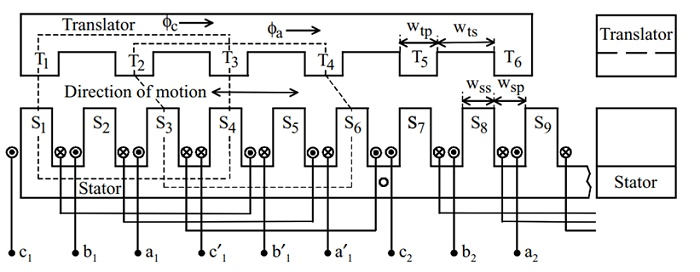Figure 1 - Typical linear switched reluctance motor structure 

In this article, both 2D and 3D electromagnetic simulation of linear switched reluctance motor examples are used. Magnetic fields, inductance and force results are computed and compared to references.

## Longitudinal Linear Switched Reluctance Motor

Figure 2 shows a 2D geometry of a longitudinal linear switched reluctance motor . Both translator and stator are made of steel 1008. It is characterized by a high magnetic field saturation value. The 234-turns stranded coil is made of copper. During the simulation, the axial force along x-axis will be computed at different translator positions and current magnitudes. The inductance results will be also calculated.

This model represents an axial invariant geometry, hence a 2D simulation can be used without compromising on accuracy. A parametric sweep analysis is performed with EMWorks2D.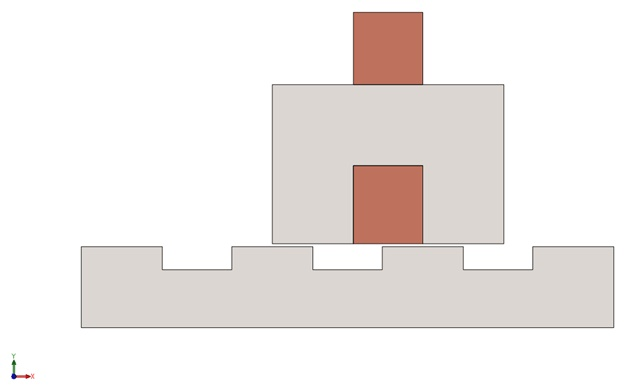Figure 2 - 2D geometry of the linear switched reluctance motor

The excitation current waveform is shown in the figure below. The simulation is established with same current waveform and different magnitudes and various translator positions. The displacement constant of the studied motor is 52mm.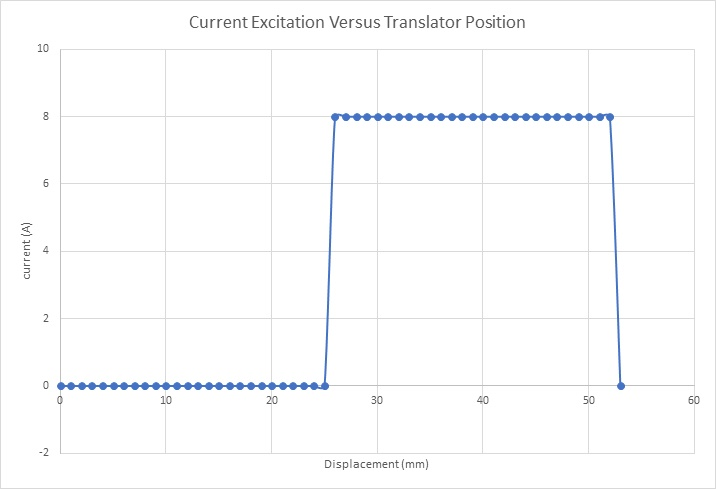Figure 3 - Excitation current waveform

## Results

2D Magnetic static results are generated of all the translator positions using parametrization study of EMWorks2D. Figure 4a) shows the magnetic flux density at an unaligned position (26mm) and an excitation of 8A. High spots of magnetic flux density of around 2T are noticed at the translator and stator poles. The blue color covers the most area of the model which represents the field values less than 0.55T. The magnetic reluctance is maximum at this position. Figure 4b) contains a plot of the magnetic flux density at the aligned position 52mm with the same excitation current. At this position, the magnetic field is much higher in a larger area of the motor model. The magnetic field is mainly between the values of 0.6T and 1.66T. This position is characterized by its minimum magnetic reluctance. An animation of the translator displacement from unaligned to aligned position is shown in Figure 5.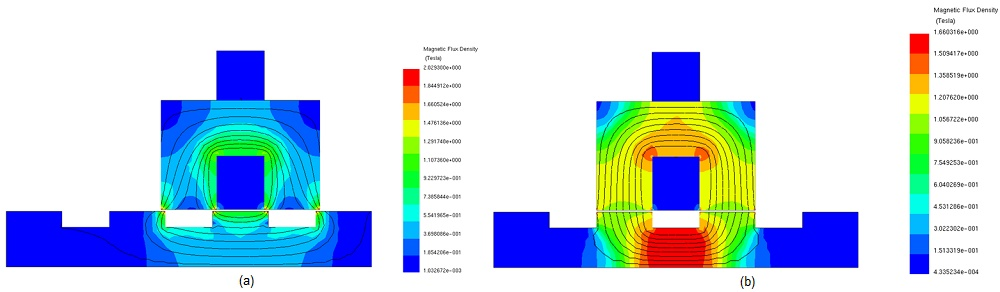Figure 4 -Magnetic field plot, a) unaligned position, b) aligned position

An animation of the translator displacement from unaligned to aligned position is shown in Figure 5.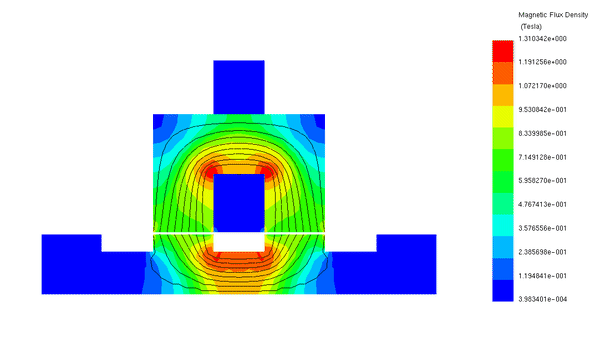Figure 5 - Animation of the magnetic flux (5A)

Figure 6 displays the 2D inductance results versus the translator displacement between two aligned positions (0 and 52 mm). The inductance is maximum (28mH) at both aligned positions. This is due to the low magnetic reluctance at these positions as mentioned above. Unlike in the aligned position, the unaligned inductance is at its lowest value (13.66 mH) because of the maximum magnetic reluctance reached at this position. Figure 7 contains the x-component of the force results versus the translator movement at various DC currents. The force results behave identically for the different excitations. Initially zero, the force starts to increase from the unaligned position after turning on the excitation. It continues to rise to its peak value of 24N at 40mm (8A). The force decreases from its peak until becoming null in the aligned position (52mm). The high values of the linear SRM force are reached in the partial overlapping positions which are located between the unaligned and aligned positions (no overlapping and full state overlapping positions). Both Figure 6 and Figure 7 demonstrates a good agreement of EMWorks2D and References ,.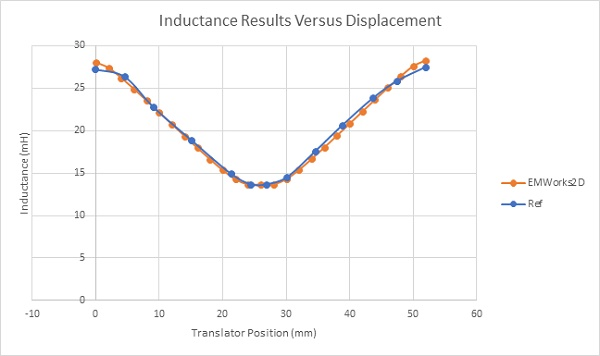Figure 6 - Inductance Results at 8A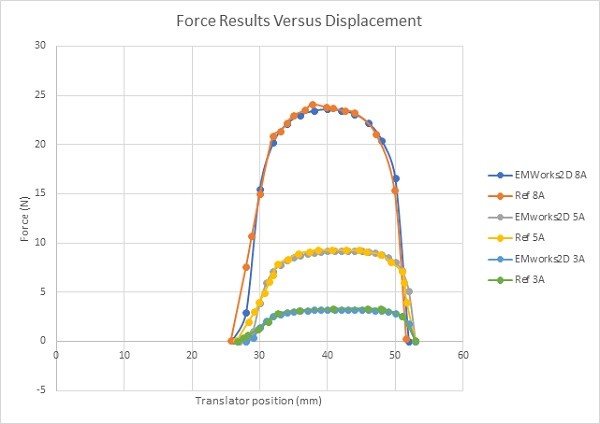Figure 7 - Force results at different current excitations

Transverse Linear Switched Reluctance MotorIn this section, a transverse linear switched reluctance motor is studied using EMS which is a 3D electromagnetic simulation software. SOLIDWORKS 3D CAD model of the simulated model is shown in Figure 8.  The same materials and coil settings are used for this example as in the previous study.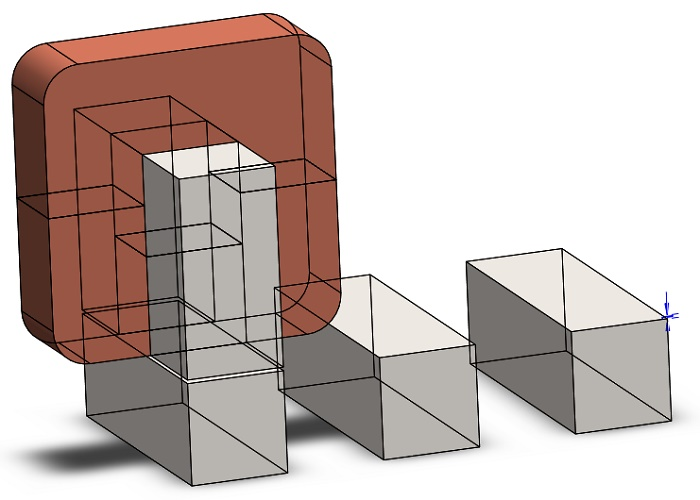Figure 8 - 3D SOLIDWORKS geometry of the transverse linear switched reluctance motor

The magnetic flux density of the motor is shown at an unaligned and aligned positions in Figure 9a and Figure 9b, respectively. The same above interpretations can be extracted from these figures because both longitudinal and transverse linear switched reluctance motors have the same operating principle.

An animation of the magnetic flux versus position is displayed In Figure 10.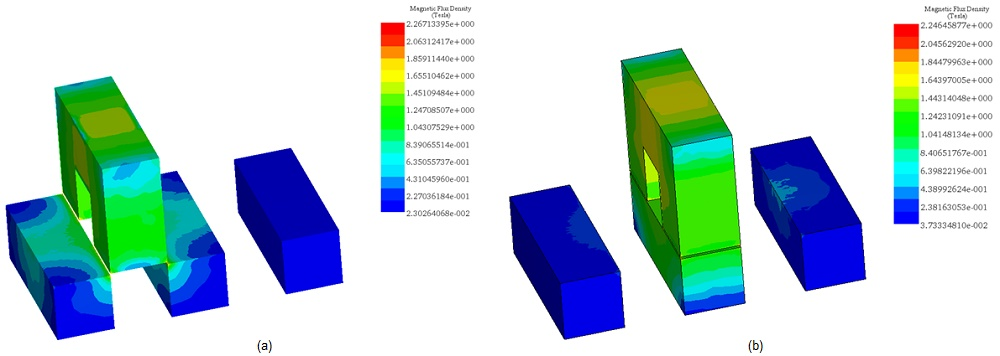Figure 9 - Magnetic flux density plot, a) at an unaligned position b) at an aligned position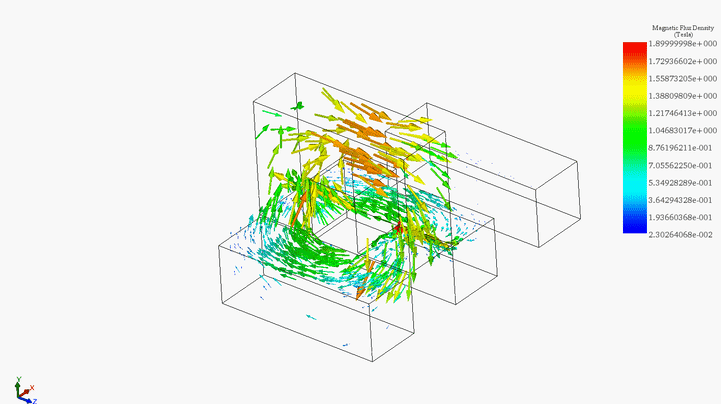Figure 10 - Animation of the magnetic field vector plot

Figure 11 contains the inductance results of the transverse LSRM versus the translator displacement. It shows that the inductance curve has two peak values at the aligned positions due the minimum magnetic reluctance of the machine. Contrarily, the inductance is minimum when the magnetic reluctance is maximum at the unaligned position. Figure 12 contains the x-component of the reluctance force at 8A. It begins to rise at 27 mm, reaches its maximum values from 20 N up 26 N at the displacement interval of [30, 50mm] and finally it becomes null again at 54mm.

The maximum force generated by the transverse linear switched reluctance motor (26.3N) is higher than the longitudinal linear switched reluctance motor. Thus, the transverse machine is preferred because it develops higher force density.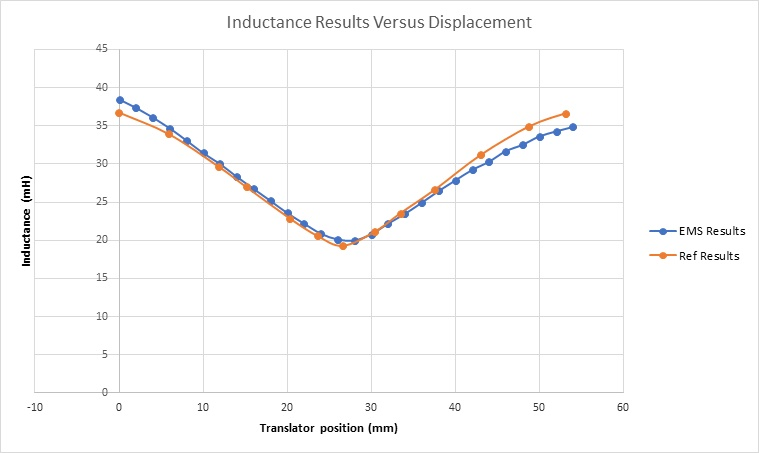Figure 11 - Inductance results versus translator positions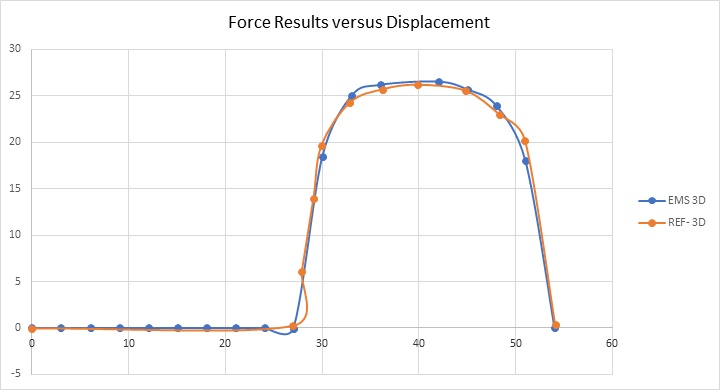Figure 12 - Force results versus translator positions

Comparison of Longitudinal and transverse switched reluctance motors Average force of both longitudinal and transverse switched reluctance motors is computed using the following formula ,:The average force of the transverse switched reluctance motor, which is about 10.28N, is higher than the average force of longitudinal switched reluctance motor which is around 8.35N. Thus, the transverse machine develops higher force density than longitudinal machine. However, longitudinal structure represents an economic machine since it needs less steel for the stator .

## Conclusion

EMWorks 2D and EMS 3D electromagnetic simulation tools were used in this example to analyze two different topologies of linear switched reluctance motor. Static results of magnetic flux, inductance and reluctance force are computed and validated with references results. Both 2D and 3D FEM simulations demonstrated their good capabilities to generate accurate results. This can help engineers to build highly efficient linear switched reluctance motor by optimizing many criteria such as the air gap. Since SRM uses the principle of attraction-repulsion force of the stator and rotor, hence a lower air gap surface between stator and rotor teeth increases the force density. However, this occurs to a higher radial pull due to the strong flux lines and thereby more torque ripples. A double-sided linear switched reluctance was developed to overcome this issue.

## References

 J Corda and E Skopljak. Linear switched reluctance actuator. In Electrical Machines and Drives, 1993. Sixth International Conference on, pages 535–539. IET, 1993.
 R. Krishnan. RELUCTANCE MOTOR DRIVES. IEEE The Bradley Department of Electrical and Computer Engineering Fellow, Center for Organizational and Technological Advancement (COTA) Virginia Tech, Blacksburg, Virginia
 S.Darabi, M.Ardebili. Analysis of Linear Switched Reluctance Motor with Longitudinal Flux Linkage Using 2D-FEM Compared to 3D-FEM. Department of Elec. Eng. K.N.Toosi University of Technology K.N.Toosi University of Technology, KNTU Tehran, Iran. 2011 2nd Power Electronics, Drive Systems and Technologies Conference
 Saeed Darabi, Yousef Alinejad Beromi, and Hamid Reza Izadfar. Comparison of Two Common Configurations of LSRM: Transverse Flux and Longitudinal Flux. Dept. of Elec. & Comp. Eng. Semnan University Semnan, Iran. 2012 International Conference and Exposition on Electrical and Power Engineering (EPE 2012), 25-27 October, Iasi, Romania